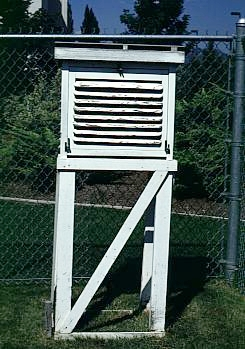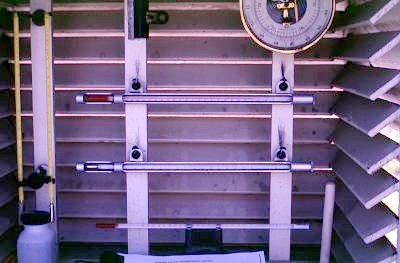PhysicalGeography.net | FUNDAMENTALS eBOOK

CHAPTER 7: Introduction to the Atmosphere

# (k). The Concept of Temperature

Temperature and Heat

Temperature and heat are not the same phenomenon. Temperature is a measure of the intensity or degree of hotness in a body. Technically, it is determined by getting the average speed of a body's molecules. Heat is a measure of the quantity of heat energy present in a body. The spatial distribution of temperature in a body determines heat flow. Heat always flows from warmer to colder areas.

The heat held in a object depends not only on its temperature but also its mass. For example, let us compare the heating of two different masses of water (Table 7k-1). In this example, one mass has a weight of 5 grams, while the other is 25 grams. If the temperature of both masses is raised from 20 to 25° Celsius, the larger mass of water will require five times more heat energy for this increase in temperature. This larger mass would also contain contain 5 times more stored heat energy.

 Table 7k-1: Heat energy required to raise two different quantities of water 5° Celsius.

 Mass of the Water Starting Temperature Ending Temperature Heat Required 5 grams 20° Celsius 25° Celsius 25 Calories of Heat 25 grams 20° Celsius 25° Celsius 125 Calories of Heat

Temperature Scales

A number of measurement scales have been invented to measure temperature. Table 7k-2 describes important temperatures for the three dominant scales in use today.

 Table 7k-2: Temperature of absolute zero, the ice point of water, and the stream point of water using various temperature measurement scales.

 Measurement Scale Steam Point of Water Ice Point of Water Absolute Zero Fahrenheit 212 32 -460 Celsius 100 0 -273 Kelvin 373 273 0

The most commonly used scale for measuring temperature is the Celsius system. The Celsius scale was developed in 1742 by the Swedish astronomer Anders Celsius. In this system, the melting point of ice was given a value of 0, the boiling point of water is 100, and absolute zero is -273. The Fahrenheit system is a temperature scale that is used exclusively in the United States. This system was created by German physicist Gabriel Fahrenheit in 1714. In this scale, the melting point of ice has a value of 32, water boils at 212, and absolute zero has a temperature of -460. The Kelvin scale was proposed by British physicist Lord Kelvin in 1848. This system is often used by scientists because its temperature readings begin at absolute zero and due to the fact that this scale is proportional to the amount of heat energy found in an object. The Kelvin scale assigns a value of 273 for the melting temperature of ice, while the boiling point of water occurs at 373.

Measurement of Air Temperature

A thermometer is a device that is used to measure temperature. Thermometers consist of a sealed hollow glass tube filled with some type of liquid. Thermometers measure temperature by the change in the volume of the liquid as it responds to the addition or loss of heat energy from the environment immediately outside its surface. When heat is added, the liquid inside the thermometer expands. Cooling cause the liquid to contract. Meteorological thermometers are often filled with either alcohol or mercury. Alcohol thermometers are favored in very cold environments because of this liquid's low freezing point (-112° Celsius).

By international agreement, the nations of the world have decided to measure temperature in a similar fashion. This standardization is important for the accurate generation of weather maps and forecasts, both of which depend on having data determined in a uniform way. Weather stations worldwide try to determine minimum and maximum temperatures for each and every day. By averaging these two values, daily mean temperatures are also calculated. Many stations also take temperature readings on the hour. Temperature measurements are determined by thermometers designed and approved by the World Meteorological Organization (see http://www.wmo.ch). These instruments are housed in specially designed instrument shelters that allow for the standardization of measurements taken anywhere on the Earth (Figure 7k-1 and Figure 7k-2).Figure 7k-1: Well ventilated instrument shelters are used to protect thermometers from precipitation, direct Sun, and other physical elements. Construction standardization of these shelters, by international agreement, guarantees that measurements are comparable in any of the over 15,000 weather stations found worldwide.Figure 7k-2: Thermometers found inside the instrument shelter are mounted approximate 1.5 meters above the ground surface. The top thermometer contains alcohol and is used to determine daily minimum temperatures. The lower thermometer uses mercury to determine the daily maximum temperature.

Study Guide